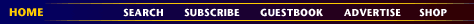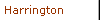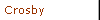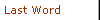Six Sigma and BeyondThomas PyzdekSelecting Winning ProjectsSoftware helps select the best projects.In a previous column I discussed how Six Sigma projects should be selected using the theory of constraints (TOC). After attempting to do so, most discover yet another constraint: money. In most organizations there are more opportunities for improvement than one can afford to pursue. If it isn't money, some other resource will be in short supply, such as talent. And as if that weren't bad enough, the task is further complicated by uncertainty of the payoff from the projects and their probability of success.

An exciting computer software product known as Crystal Ball Pro by Decisioneering makes it possible to select winning projects by factoring in all of the relevant factors. It does so by simulating various scenarios thousands of times, then choosing those that perform best.

For example, the research and development group of a major public utility has identified eight possible Six Sigma projects. A net present value analysis has computed: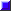The expected revenue for each project, if it's successfulIts estimated probability of successIts required initial investment

Using these figures, the finance manager has computed the expected return and the expected profit for each project. Unfortunately, the available budget is only \$2 million, and selecting all projects would require a total initial investment of \$2.8 million. Thus, the objective is to determine which projects will maximize the total expected profit while staying within the budget limitation. Complicating this decision is the fact that both the expected revenue and success rates are highly uncertain. Figure 1 shows a spreadsheet model for this problem.The decision variables in column H are binary; that is, they can only assume the values zero (do not fund the project) and one (fund the project.) The assumption variables are in the "Expected Revenue" and "Success Rate" columns. Crystal Ball Pro will use simulation to evaluate a range of values for these two columns. The total profit, shown in cell G19, is a forecast variable whose values depend on the assumption and decision variables. The idea is to find the combination of projects (determined by the decision variables) that maximize total profit, taking into account the variation in expected revenue and the probability of success.

The project selection spreadsheet isn't quite good enough given that the number of possible sets of projects is too large to identify by trial-and-error. Crystal Ball Pro can help here too. It includes a
program, called OptQuest, which will perform a search to find the optimal package of projects (see Figure 2).

Figure 2: Progress Toward a SolutionThe best solution OptQuest found (in a search that I limited to 10 minutes) is to fund all projects except 3 and 5 (see Figure 3). The expected net profit is \$1.54 million. Note that the distribution of total profit includes a number of scenarios that would result in a net loss. This occurs because OptQuest was asked to find the solution that maximized expected (average) total profit, but it can limit searches to profitable software solutions too.

Figure 3: Results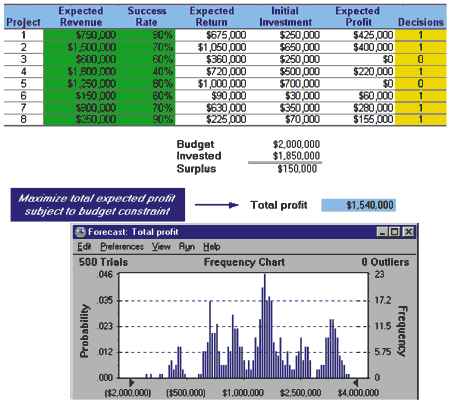Menu Level Above This Menu LeveL Menu Level BelowCopyright 2000 QCI International. All rights reserved. Quality Digest can be reached by phone at (530) 893-4095. E-mail: Click Here